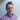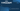Carl Rippon

# TypeScript Dictionary

November 04, 2020
typescript

This post covers different ways to strongly-type a dictionary in TypeScript. Dictionaries are sometimes referred to as a hash or a map - basically it is a collection of key-value pairs. In this post we are going to focus on dictionaries where the keys are unknown - if we know the keys then a type alias or interface can be used.

## The problem

TypeScript needs to understand the full representation of an object before it is accessed. For example, the following code is fine in JavaScript but raises a type error in TypeScript:

``````let scores = {};
scores.bill = 10; // 💥 - Property 'bill' does not exist on type '{}'``````

The following code outputs `undefined` to the console in JavaScript but raises a type error in TypeScript:

``````let scores = { bill: 10 };
console.log(scores.fred); // 💥 - Property 'fred' does not exist on type '{ bill: number; }'``````

We can use `any` in a type annotation, but then no type checking will occur on the dictionary:

``````let scores: any = {};
scores.bill = 10; // ✔️ - no type error
scores.invalidProp = true; // ✔️ - no type error``````

We want some type checking to happen but have the flexibility to add keys into the dictionary at runtime.

## Using an indexed object type annotation

We can use an indexed object type annotation as follows:

``````let scores: { [name: string]: number } = {};
scores.bill = 10; // ✔️ - no type error
scores.bill = "10"; // 💥 - Type 'string' is not assignable to type 'number'``````

Here we specify that the dictionary keys are strings and the values are numeric.

The “name” label can be anything we like. Often “key” is used:

``````let scores: { [key: string]: number } = {};
scores.bill = 10;``````

The label can’t be omitted though:

``````let scores: { [string]: number } = {};
// 💥 - 'string' only refers to a type, but is being used as a value here``````

Unfortunately we can’t restrict keys using a union type:

``````let scores: {
[name: "bill" | "bob"]: number;
} = {};
// 💥 - An index signature parameter type cannot be a union type. Consider using a mapped object type instead``````

On a more postive note, we can have more complex value types:

``````type Person = {
email: string;
rating: number;
};
let scores: { [name: string]: Person } = {};
scores.bill = {
email: "bill@somewhere.com",
rating: 9,
};
scores.bob = {
// 💥  Type '{ emailAddress: string; rating: number; }' is not assignable to type 'Person'.
rating: 9,
};``````

## Using the `Record` utility type

There is a `Record` utility type that is a little more concise than an indexed object type. It also allows the key type to be a union type.

``````let scores: Record<string, number> = {};
scores.bill = 10; // ✔️ - no type error
scores.trevor = "10"; // 💥 - Type 'string' is not assignable to type 'number'``````

We can narrow the type of the keys using a union type as follows:

``````let scores: Record<"bill" | "bob", number> = {};
scores.bill = 10; // ✔️ - no type error
scores.trevor = 10; // 💥 - Property 'trevor' does not exist on type 'Record<"bill" | "bob", number>'``````

## Using Map

A Map is a standard JavaScript feature that is useful for holding key-value pairs.

There is a corresponding TypeScript type for a `Map` called `Map`. This is a generic type that takes in the types for the key and value as parameters:

``````let scores = new Map<string, number>();
scores.set("bill", 10);
scores.set("bob", "10"); // 💥 - Argument of type 'string' is not assignable to parameter of type 'number'.``````

We can use a union type for the keys and an object type for the values as follows:

``````type Person = {
email: string;
rating: number;
};
let scores = new Map<"bill" | "bob", Person>();
scores.set("bill", {
email: "bill@somewhere.com",
rating: 9,
});``````

A benefit of `Map` is that it provides a nice API for accessing items in the object:

``````let scores = new Map<"bill" | "bob", Person>();
scores.set("bill", {
email: "bill@somewhere.com",
rating: 9,
});
scores.set("bob", {
email: "bob@somewhere.com",
rating: 9,
});
console.log(scores.has("bill")); // true

scores.forEach((person) => console.log(person));
// { "email": "bill@somewhere.com", "rating": 9 }
// { "email": "bob@somewhere.com", "rating": 9 }``````

Nice !

## Wrap up

The `Record` utility type is a concise approach to strongly typing a dictionary. If we want a nicer API around the dictionary we can use `Map`.

## Did you find this post useful?

Let me know by sharing it on Twitter.